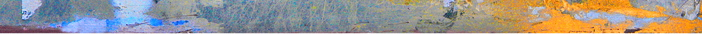ClassicalRealAnalysis.com
Mathematics textbooksHomeTCI-TableOfContents

The Calculus IntegralContents

 Preface i Note to the instructor iii Table of Contents vii 1 What you should know first 1 1.1 What is the calculus about? 1 1.2 What is an interval? 2 1.3 Sequences and series 4 1.4 Partitions 8 1.5 Continuous functions 10 1.6 Existence of maximum and minimum 21 1.7 Derivatives 23 1.8 Differentiation rules 25 1.9 Mean-value theorem 26 1.10 Lipschitz functions 33 2 The Indefinite Integral 35 2.1 An indefinite integral on an interval 35 2.2 Existence of indefinite integrals 39 2.3 Basic properties of indefinite integrals 4 2.3 Basic properties of indefinite integrals 42 3 The Definite Integral 49 3.1 Definition of the calculus integral 50 3.2 Integrability 55 3.3 Properties of the integral 58 3.4 Mean-value theorems for integrals 63 3.5 Riemann sums 64 3.6 Absolute integrability 79 3.7 Sequences and series of integrals 84 3.8 The monotone convergence theorem 97 3.9 Integration of power series 99 3.10 Applications of the integral 106 3.11 Numerical methods 114 3.12 More Exercises 119 4 Beyond the calculus integral 121 4.1 Countable sets 121 4.2 Derivatives which vanish outside of countable sets 123 4.3 Sets of measure zero 125 4.4 The Devil’s staircase 130 4.5 Functions with zero variation 132 4.6 The integral 138 4.7 Approximation by Riemann sums 142 4.8 Properties of the integral 143 4.9 The Henstock-Kurweil integral 148 4.10 The Riemann integral 149 5 Answers 153 5.1 Answers to problems 153 Index 288